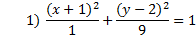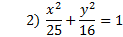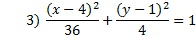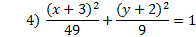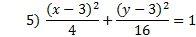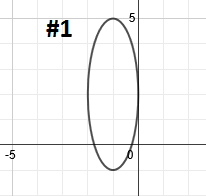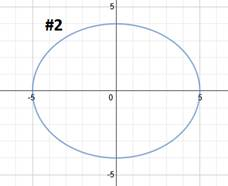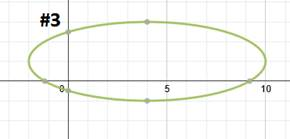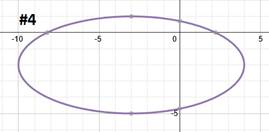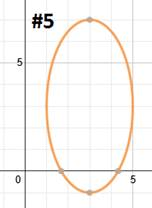# Graphing Ellipses

To graph an ellipse, you must first be able to identify the center point, whether it's horizontal or vertical, and the a and b values. These were discussed in the last lesson.

Now we will take this information and use it to graph an ellipse.

Before we do that, though, let's review the patterns: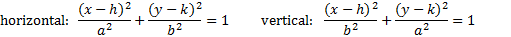To graph an ellipse:

1. Find and graph the center point.
2. Determine if the ellipse is vertical or horizontal and the a and b values.
3. Use the a and b values to plot the ends of the major and minor axis.
4. Draw in the ellipse.

Let's graph a couple, and you'll see how it works.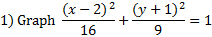First we must identify the center point, which is (2, -1). (Remember that you must take the opposite of each number inside the parenthesis.) Let's graph that point:Now we need to determine if the ellipse is vertical or horizontal. Since the larger number is under the x, we know it is horizontal. Remember to find a and b, we have to take the square roots of the denominators. So a = 4 and b = 3.

We can now use a and b to plot the ends of the major and minor axis. This parabola is horizontal and the a value (4) is the distance from the center point to the end of the major axis. Since the major axis is horizontal, we'll count right 4 and left 4 to plot its endpoints.Now we'll use the b value (3) to find the ends of the minor axis. Let's count up 3 blocks and down 3 blocks and plot the points there.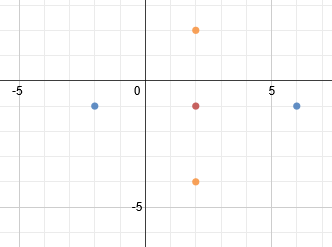To finish, we simply need to draw in the ellipse.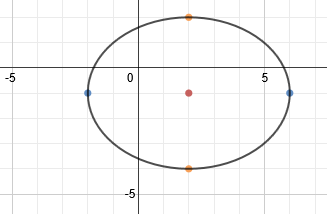Once again, the center point is not technically part of the answer, so a technical graph would look like this: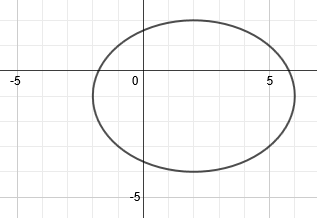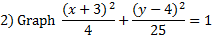First we must identify the center point, which is (-3, 4). Let's graph it: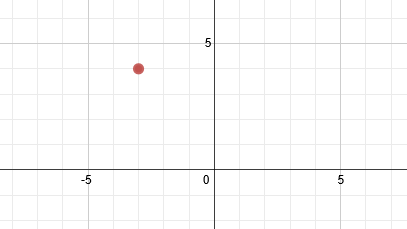Now we need to determine if the ellipse is vertical or horizontal. Since the larger number is under the y, we know it is vertical. Remember to find a and b, we have to take the square roots of the denominators. So a = 5 and b = 2.

We must now use a and b to plot the ends of the major and minor axis. This parabola is vertical and the a value (5) is the distance from the center point to the end of the major axis. Since the major axis is vertical, we'll count up 5 and down 5 to plot its endpoints.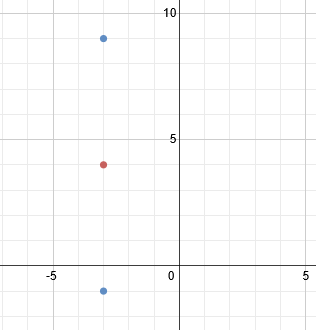Now we'll use the b value (2) to find the ends of the minor axis. Let's count left 2 blocks and right 2 blocks and plot the points there.To finish, we simply need to draw in the ellipse.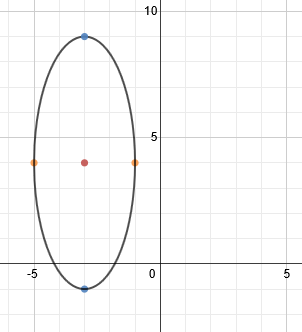Practice: Graph each ellipse.